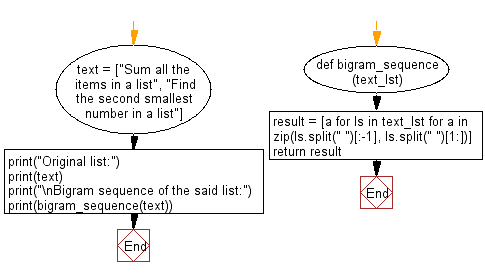﻿ Python: Bigrams of words in a given list of strings - w3resource# Python: Bigrams of words in a given list of strings

## Python List: Exercise - 184 with Solution

Write a Python program to form Bigrams of words in a given list of strings.

From Wikipedia:
A bigram or digram is a sequence of two adjacent elements from a string of tokens, which are typically letters, syllables, or words. A bigram is an n-gram for n=2. The frequency distribution of every bigram in a string is commonly used for simple statistical analysis of text in many applications, including in computational linguistics, cryptography, speech recognition, and so on.

Sample Solution:

Python Code:

``````def bigram_sequence(text_lst):
result = [a for ls in text_lst for a in zip(ls.split(" ")[:-1], ls.split(" ")[1:])]
return result
text = ["Sum all the items in a list", "Find the second smallest number in a list"]
print("Original list:")
print(text)
print("\nBigram sequence of the said list:")
print(bigram_sequence(text))
```
```

Sample Output:

```Original list:
['Sum all the items in a list', 'Find the second smallest number in a list']

Bigram sequence of the said list:
[('Sum', 'all'), ('all', 'the'), ('the', 'items'), ('items', 'in'), ('in', 'a'), ('a', 'list'), ('Find', 'the'), ('the', 'second'), ('second', 'smallest'), ('smallest', 'number'), ('number', 'in'), ('in', 'a'), ('a', 'list')]
```

Flowchart:## Visualize Python code execution:

The following tool visualize what the computer is doing step-by-step as it executes the said program:

Python Code Editor:

Have another way to solve this solution? Contribute your code (and comments) through Disqus.

What is the difficulty level of this exercise?

Test your Python skills with w3resource's quiz

﻿

## Python: Tips of the Day

```print(2_000_000)
```2000000